Home
Hostname: page-component-66d7dfc8f5-6zwsx Total loading time: 0.473 Render date: 2023-02-08T16:53:57.561Z Has data issue: true Feature Flags: { "useRatesEcommerce": false } hasContentIssue true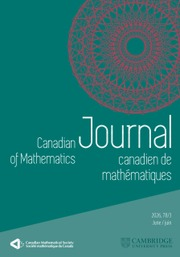Canadian Journal of Mathematics

# Unbounded Fredholm Modules and Spectral Flow

Published online by Cambridge University Press:  20 November 2018

## Abstract

HTML view is not available for this content. However, as you have access to this content, a full PDF is available via the ‘Save PDF’ action button.

An odd unbounded (respectively,$p$-summable) Fredholm module for a unital Banach$*$-algebra,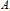$A$, is a pair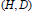$(H,D)$ where$A$ is represented on the Hilbert space,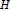$H$, and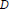$D$ is an unbounded self-adjoint operator on$H$ satisfying:

(1)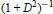${{(1+{{D}^{2}})}^{-1}}$ is compact (respectively, Trace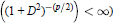$\left( {{\left( 1+{{D}^{2}} \right)}^{-(p/2)}} \right)<\infty$, and

(2)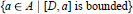$\{a\in A|\,\,[D,a]\,\,\text{is}\,\,\text{bounded}\}\text{ }$ is a dense$*$- subalgebra of$A$.

If$u$ is a unitary in the dense$*$-subalgebra mentioned in (2) then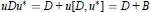$$uD{{u}^{*}}=D+u[D,{{u}^{*}}]=D+B$$

where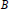$B$ is a bounded self-adjoint operator. The path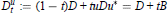$$D_{t}^{u}:=(1-t)D+tuD{{u}^{*}}=D+tB$$

is a “continuous” path of unbounded self-adjoint “Fredholm” operators. More precisely, we show that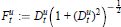$$F_{t}^{u}:=D_{t}^{u}{{\left( 1+{{\left( D_{t}^{u} \right)}^{2}} \right)}^{-\frac{1}{2}}}$$

is a norm-continuous path of (bounded) self-adjoint Fredholm operators. The spectral flow of this path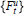$\left\{ F_{t}^{u} \right\}$ (or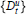$\{D_{t}^{u}\}$) is roughly speaking the net number of eigenvalues that pass through 0 in the positive direction as$t$ runs from 0 to 1. This integer,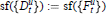$$\text{sf}\left( \left\{ D_{t}^{u} \right\} \right):=\text{sf}\left( \left\{ F_{t}^{u} \right\} \right),$$

recovers the pairing of the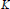$K$-homology class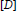$[D]$ with the$K$-theory class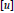$[u]$.

We use I.M. Singer's idea (as did E. Getzler in the$\theta$-summable case) to consider the operator$B$ as a parameter in the Banach manifold,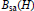${{B}_{\text{sa}}}\left( H \right)$, so that spectral flow can be exhibited as the integral of a closed 1-form on this manifold. Now, for$B$ in our manifold, any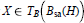$X\,\in \,{{T}_{B}}({{B}_{\text{sa}}}(H))$ is given by an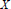$X$ in${{B}_{\text{sa}}}(H)$ as the derivative at$B$ along the curve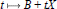$t\,\mapsto \,B\,+\,tX$ in the manifold. Then we show that for$m$ a sufficiently large half-integer: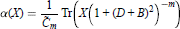$$\alpha (X)=\frac{1}{{{{\tilde{C}}}_{m}}}\text{Tr}\left( X{{\left( 1+{{\left( D+B \right)}^{2}} \right)}^{-m}} \right)$$

is a closed 1-form. For any piecewise smooth path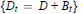$\left\{ {{D}_{t}}=D+{{B}_{t}} \right\}$ with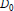${{D}_{0}}$ and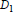${{D}_{1}}$unitarily equivalent we show that$$\text{sf}\left( \left\{ {{D}_{t}} \right\} \right)=\frac{1}{{{{\tilde{C}}}_{m}}}\int_{\text{0}}^{\text{1}}{\text{T}}\text{r}\left( \frac{d}{dt}\left( {{D}_{t}} \right){{\left( 1+D_{1}^{2} \right)}^{-m}} \right)dt$$

the integral of the 1-form$\alpha$. If${{D}_{0}}$ and${{D}_{1}}$ are not unitarily equivalent, wemust add a pair of correction terms to the right-hand side. We also prove a bounded finitely summable version of the form: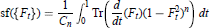$$\text{sf}\left( \left\{ {{F}_{t}} \right\} \right)=\frac{1}{{{C}_{n}}}\int_{\text{0}}^{\text{1}}{\text{T}}\text{r}\left( \frac{d}{dt}\left( {{F}_{t}} \right){{\left( 1-{{F}_{t}}^{2} \right)}^{n}} \right)dt$$

for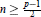$n\ge \frac{p-1}{2}$ an integer. The unbounded case is proved by reducing to the bounded case via the map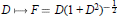$D\mapsto F=D{{(1+{{D}^{2}})}^{-\frac{1}{2}}}$ We prove simultaneously a type II version of our results.

## Keywords

Type
Research Article
Information
Canadian Journal of Mathematics , 01 August 1998 , pp. 673 - 718

## References

[APS] Atiyah, M. F., Patodi, V. K. and Singer, I. M., Spectral asymmetry and Riemannian geometry. III. Math. Proc. Cambridge Philos. Soc. 79(1976), 7199.Google Scholar
[ASS] Avron, J., Seiler, R. and Simon, B., The index of a pair of projections. J. Funct. Anal. 120(1994), 220237.Google Scholar
[BF] Booß-Bavnbek, B. and Furutani, K., The Maslov Index: A Functional Analytic Definition and the Spectral Flow Formula. (1995), preprint.Google Scholar
[BW] B., Booß-Bavnbek and Wojciechowski, K. P., Elliptic Boundary Problems for Dirac Operators. Birkhäuser, Boston, Basel, Berlin, 1993.Google Scholar
[B1] Breuer, M., Fredholm theories in von Neumann Algebras, I. Math. Ann. 178(1968), 243254.Google Scholar
[B2] Breuer, M., Fredholm theories in von Neumann Algebras, II. Math. Ann. 180(1969), 313325.Google Scholar
[CP] Carey, A. L. and Phillips, J., Algebras almost commuting with Clifford algebras in a II∞ factor. K-Theory 4(1991), 445478.Google Scholar
[C1] Connes, A., Noncommutative differential geometry. Publ. Inst. Hautes Études Sci. Publ. Math. 62(1985), 41144.Google Scholar
[CMX] Curto, R., Muhly, P. S. and Xia, J., Toeplitz operators on flows. J. Funct. Anal. 93(1990), 391450 Google Scholar
[D] Dixmier, J., Formes Linéaires sur un Anneau d’Opérateurs. Bull. Soc. Math. France 81(1953), 939.Google Scholar
[DHK] Douglas, R. G., S.Hurder and Kaminker, J., Cyclic cocycles, renormalization and eta-invariants. Invent. Math. 103(1991), 101179.Google Scholar
[DS] Dunford, N. and Schwartz, J. T., Linear Operators, Part II. Wiley, New York, London, 1963.Google Scholar
[FK] Fack, T. and Kosaki, H., Generalized s-numbers of τ-measurable operators. Pacific J. Math. 123(1986), 269300.Google Scholar
[G] Getzler, E., The odd Chern character in cyclic homology and spectral flow. Topology 32(1993), 489507.Google Scholar
[H] Hurder, S., Eta invariants and the odd index theorem for coverings. Contemporary Math. (2) 105(1990), 4782.Google Scholar
[K] Kato, T., Perturbation Theory for Linear Operators. Springer-Verlag, New York, 1966.Google Scholar
[Kam] Kaminker, J., Operator algebraic invariants for elliptic operators. Proc. Symp. PureMath. (I) 51(1990), 307314.Google Scholar
[L] Lesch, M., On the index of the infinitesimal generator of a flow. J. Operator Theory 26(1991), 7392.Google Scholar
[M] Mathai, V., Spectral flow, eta invariants and von Neumann algebras. J. Funct.Anal. 109(1992), 442456.Google Scholar
[Ped] Pedersen, G. K., C*-Algebras and Their Automorphism Groups. Academic Press, London, New York, San Francisco, 1979.Google Scholar
[Per] Perera, V. S., Real valued spectral flow. Contemporary Math. 185(1993), 307318.Google Scholar
[P2] Phillips, J., Spectral flow in type I and II factors—A new approach. Fields Inst. Comm., Cyclic Cohomology & Noncommutative Geometry, 17(1997), 137153.Google Scholar
[PR] Phillips, J. and Raeburn, I. F., An index theorem for Toeplitz operators with noncommutative symbol space. J. Funct. Anal. 120(1994), 239263.Google Scholar
[RS] Reed, M. and Simon, B., Methods of Modern Mathematical Physics IV: Analysis of Operators. Academic Press, New York, San Francisco, London, 1978.Google Scholar
[S] Spivak, M., A Comprehensive Introduction to Differential Geometry. vol. I, 2nd ed., Publish or Perish Inc., Berkeley, 1979.Google Scholar
[Si] Singer, I. M., Eigenvalues of the Laplacian and Invariants of Manifolds. Proc. International Congress I, Vancouver, 1974. 187200.Google Scholar
[Su] Sukochev, F. A., Perturbation Estimates for a Certain Operator-Valued Function. Flinders University, 1998. preprint.Google ScholarYou have Access
48
Cited by

# Save article to Kindle

Note you can select to save to either the @free.kindle.com or @kindle.com variations. ‘@free.kindle.com’ emails are free but can only be saved to your device when it is connected to wi-fi. ‘@kindle.com’ emails can be delivered even when you are not connected to wi-fi, but note that service fees apply.

Find out more about the Kindle Personal Document Service.

Unbounded Fredholm Modules and Spectral Flow
Available formats
×

# Save article to Dropbox

To save this article to your Dropbox account, please select one or more formats and confirm that you agree to abide by our usage policies. If this is the first time you used this feature, you will be asked to authorise Cambridge Core to connect with your Dropbox account. Find out more about saving content to Dropbox.

Unbounded Fredholm Modules and Spectral Flow
Available formats
×

# Save article to Google Drive

To save this article to your Google Drive account, please select one or more formats and confirm that you agree to abide by our usage policies. If this is the first time you used this feature, you will be asked to authorise Cambridge Core to connect with your Google Drive account. Find out more about saving content to Google Drive.

Unbounded Fredholm Modules and Spectral Flow
Available formats
×
×